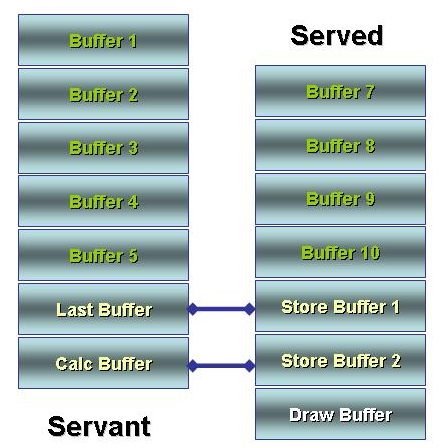# DECEMA Indicator

Hi folks!

Well, this article must called "How to work with number of buffers greater than the olny 8 buffers that the MQL4 supports".
One of my friends (JoZo) asked me to convert a piece of VTTrader code into MQL4! The indicator is DECEMA indicator and this is the code:

EMA1:= mov(pr.pe.E);
EMA2:= mov(EMA1,pe,E);
EMA3 := mov(EMA2,pe,E);
EMA4 := mov(EMA3,pe,E);
EMA5 := mov(EMA4,pe,E);
EMA6 := mov(EMA5,pe,E);
EMAT := mov(EMA6,pe,E);
EMAS := mov(EMA7,pe,E);
EMA9 := mov(EMA8,pe,E);
EMA 10:= mov(EMA9,pe,E);

DECEMA := (10 * EMA 1)-(45 * EMA2)+(120*EMA3)-(210 * EMA4)+(252 * EMA5)- (210* EMA6)+(120*EMA7)-(45 *EMA8)+(10* EMA9)-EMA 10;

I told him "It's a very simple formula but it's not transferable to MQL4 because it uses 11 buffers for calculation and the maximum number of buffer the MQL4 use are 8 buffers".

But what does it mean? It means there is a lack of flexibility in MQL4 and it's a big shame for me and all of MQL4 developers to keep the silence!

I decided to overcome the maximum buffer that MQL4 could use by splitting the DECEMA indicator to 2 indicators; The Served indicator (The DECEMA indicator itself) and the servant indicator (DECEMA-a indicator)

What's the idea behind Served and Servant indicators?

I'm using the servant indicator to hold the half of calculation buffers of DECEMA indicators (6 indicators)! And the served indicator has to give me another 2 buffers as "Connectors" to the served indicator.
One of these connectors is a holder of the last buffer of the calculation to use it in the served indicator to continue the formula. And the second connector the half of calculation of the DECEMA formula:

(10 * EMA 1)-(45 * EMA2)+(120*EMA3)-(210 * EMA4)+(252 * EMA5)- (210* EMA6)

Which we use it in the served indicator to continue the DECEMA formula as you will see!
The served indicator will load these connectors by using the iCustom function into 2 store buffers and continue the calculation of the DECEMA and finally draws the DECEMA:

DrawBuffer[shift] = StoreBuffer2[shift] + (120*Buffer7[shift])-(45*Buffer8[shift])+(10*Buffer9[shift])- Buffer10[shift];